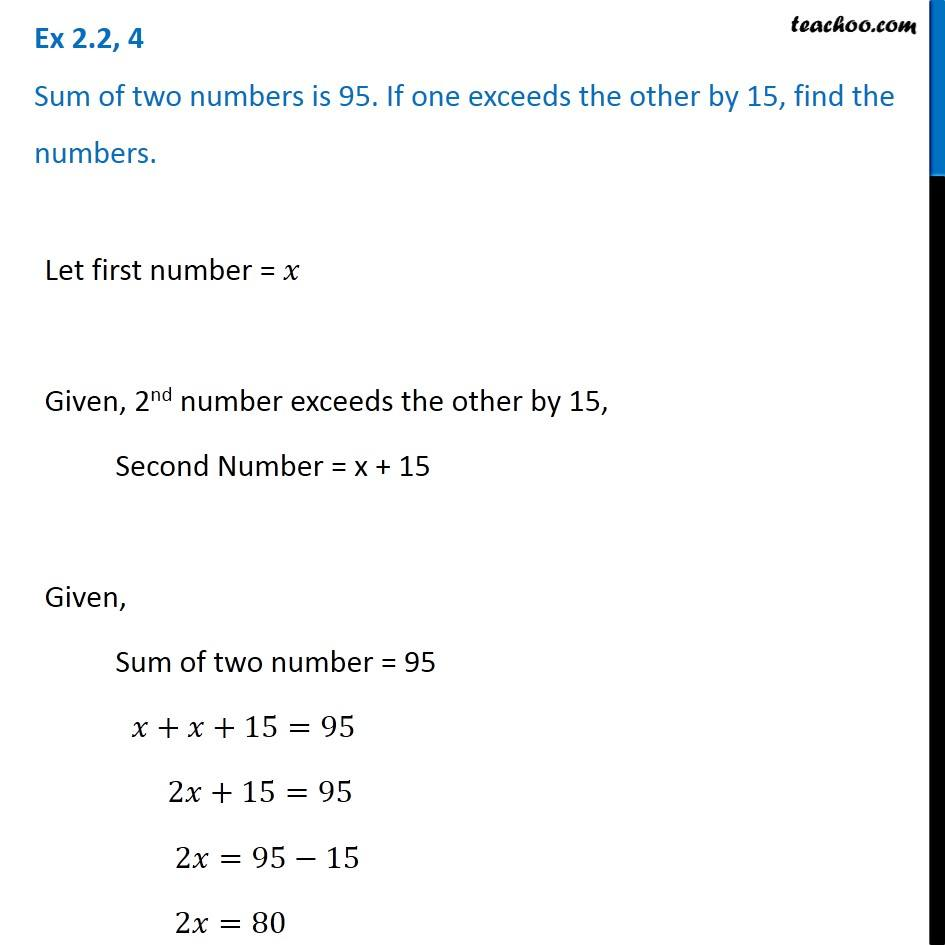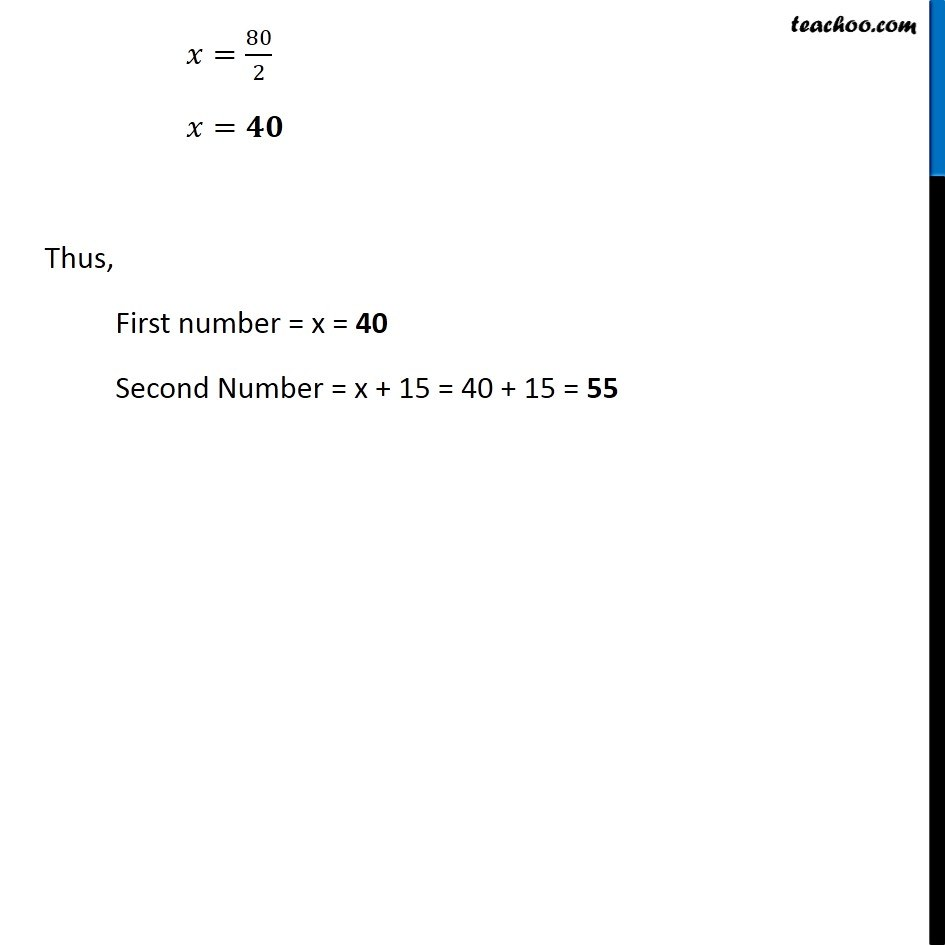1. Chapter 2 Class 8 Linear Equations in One Variable
2. Concept wise
3. Forming Linear Equations - Adding subtracting numbers

Transcript

Ex 2.2, 4 Sum of two numbers is 95. If one exceeds the other by 15, find the numbers.Let first number = 𝑥 Given, 2nd number exceeds the other by 15, Second Number = x + 15 Given, Sum of two number = 95 𝑥+𝑥+15=95 2𝑥+15=95 2𝑥=95−15 2𝑥=80 𝑥=80/2 𝑥=𝟒𝟎 Thus, First number = x = 40 Second Number = x + 15 = 40 + 15 = 55